[Contents]

Series RLC Circuit
John Denker

## 1  Derivation – Grounded Capacitor

### 1.1  Basic Formulas

Consider the RLC circuit in figure 1. There are at least two ways of thinking about it.

• One way is to treat it as a real (noisy) resistor Rx in series with an inductor and capacitor.
• Another way is to treat it as an ideal noise source VN driving a filter consisting of an ideal (noiseless) resistor R in series with an inductor and capacitor. This has the slight drawback that it requires us to talk about things that are not directly observable, but overall it is easier to analyze, so we will use this viewpoint in most of what follows.Figure 1: Series RLC Circuit, Grounded Capacitor

We start by applying Ohm’s law for complex impedances in series. The input to the filter is V0 which we will set equal to VN. The phasor current is:

I0 =
V0
R + iωL +
 1 iωC
(1)

Note that the reactive parts of the impedance add to zero at the corner frequency. (In contrast, for a parallel RLC circuit, the reactive parts would combine in parallel to make an infinite impedance at the corner frequency.)

At the moment we are not interested in the phase, so we focus attention on the magnitude of the current. Compare eq 12.3.8 in reference 1.

|I0| =
|V0
R2 + (ωL −
 1 ωC
)2 ]½
(2)

The magnitude of voltage across the capacitor is:

|VC| = |I0| |ZC|
=
|V0
ωC [ R2 + (ωL −
 1 ωC
)2 ]½
=
|V0
[ ω2C2R2 + (ω2LC −
 ωC ωC
)2 ]½
=
 |V0| [ ω2τ2 + (ω2/ω02 − 1)2 ]½
(3)

where

ω0 :=
 1/LC
the corner frequency
τ := RC    the RC time constant
(4)

Note that 1/RC has dimensions of frequency. This has important but non-obvious physical significance, as discussed in section 1.6.

The corner frequency is the intersection of the asymptotes. The true resonant frequency, as defined by the position of the peak, is not quite the same, although it converges to the corner frequency in the high-Q limit. See equation 14 and equation 28.

The magnitude of the gain squared is:

|G|2 = |VC/V0|2
=
 1 ω2τ2 + (ω2/ω02 − 1)2
=
 1 (1/Q2) ω2/ω02 + (ω2/ω02 − 1)2
jsd preferred form
=
 ω04 ω2τ2ω04 + (ω2 − ω02)2
=
 ω04 ω2δ2 + (ω2 − ω02)2
another widely-used form
(5)

where δ is the R/L bandwidth:

 δ2 := τ2ω4 = R2C2/L2C2 = R2/L2 δ = R/L
(6)

This gives us one possible notion of bandwidth. See section 1.6 for a discussion of this and other frequency-like quantities.

The quality factor Q can be expressed in a variety of useful forms:

Q :=
 1 RCω0

= ω0 L/R
=
(1/R
 L/C

= Z0 / R
(7)

and the reactive reference impedance is:

Z0 :=
 L/C
(8)

We can equally well write things in terms of the circular (not angular) frequency. Circular frequency can be measured in units of hertz (not radians per second). That gives us:

|G|2 =
 1 (1/Q2) f2/f02 + (f2/f02 − 1)2
(9)

We can also write things in terms of the normalized frequency. We define:

ϕ :=
 f f0
=
 ω ω0

(10)

whereupon:

|G|2 =
 1 (1/Q2) ϕ2 + (ϕ2 − 1)2

=
 1 (ϕ2)2 − (2 − 1/Q2) ϕ2 + 1
(11)

Continuing down the road toward more streamlined expressions, we can write things in terms of frequency squared. We define:

 ψ := ϕ2
(12)

whereupon:

|G|2 =
 1 (1/Q2) ψ + (ψ − 1)2
(13a)
=
 1 ψ2 − (2 − 1/Q2) ψ + 1
(13b)
=
 1 1/|G|2peak + (ψ − ψpeak)2
Lorentz form     (13c)

where the key parameters of the resonator are:

|G|2peak :=
 4 Q4 4 Q2 − 1

ψpeak :=
 2 Q2 − 1 2 Q2
(14)

Equation 13c and equation 14 were obtained by completing the square. Note that in equation 13c (unlike in previous expressions), the first term in the denominator in a constant, independent of frequency.

In the context of spectroscopy, equation 13c is called a Lorentzian. In particular, it is a Lorentzian function of the square of the frequency. I’m not quite sure why it’s called that. In the context of probability, the same function is called a Cauchy distribution. Furthermore, it’s essentially the same function as the “Witch” of Agnesi, and Agnesi has more than 100 years priority. On top of all that, Fermat studied the same function 100 years before Agnesi. Evidently this is yet another example of Stigler’s law of epononymy.

Expressing it in standard Lorentzian form makes it easy to pick off the abscissa and ordinate of the peak.

### 1.2  Full Width at Half Max

To find the FWHM, we set

|G|2 =

 1 1/|G|2peak + (ψ − ψpeak)2
=
 |G|2peak 2

(15)

and solve for frequency squared:

1/|G|2peak + (ψ − ψpeak)2 =
 2 |G|2peak

(ψ − ψpeak)2 =
 1 |G|2peak

ψ =
ψpeak ±
 1/|G|2peak

Δψ = 2/|G|peak
(16)

which reduces to 2/Q in the high-Q limit.

However, we are mainly interested in the frequency ϕ (not frequency squared), so we write

 ϕ12 = ψpeak − 1/|G|peak ϕ22 = ψpeak + 1/|G|peak (ϕ2 − ϕ1) (ϕ2 + ϕ1) = 2/|G|peak Δϕ ≈ 1/|G|peak for large Q
(17)

which reduces to 1/Q in the high-Q limit. More generally:

Δϕ =
 ψpeak + 1/|G|peak
−
 ψpeak − 1/|G|peak
(for QQmin)     (18a)
=
 φ2peak + 1/|G|peak
−
 φ2peak − 1/|G|peak
(for QQmin)     (18b)
=
 1 Q
+
 1 4Q3
+ terms of order 1/Q5
(Taylor series)     (18c)

where

Qmin :=
 1 + √(1/2)
(19)

When Q is equal to Qmin, the height of the peak is 2. For smaller Q values, the concept of a “peak” with a “FWHM” goes out the window. You can still define a notion of “width” but the meaning is different.

Not coincidentally, the radius of convergence of the Taylor series in equation 18c is 1/Q = 1/Qmin.

### 1.3  Thermodynamics

Consider the Nyquist formula for the Johnson noise source inside a resistor. It should be written as:

 d⟨|VN|2⟩ = 4 kT R df
(20)

where the brackets ⟨⋯⟩ denote the ensemble average. This is the voltage of the ideal noise source, deep inside the black box, where it is not directly observable. The real resistor consists of everything inside the black box in figure 1, i.e. the noise source plus the ideal noiseless resistance. The externally observable voltage VRx at the terminals of the black box depends on the noise source and on the IR voltage drop across the ideal resistance.

All too often the formula is written in the less-careful form:

 ⟨|V|2⟩ = 4 kT R B       ☠
(21)

where it requires some care to figure out what is meant by V and what is meant by the bandwidth B. If you apply equation 21 incautiously, you might imagine that you need is B, not the gain or the area under the curve. However, as usual, it pays to think about where the formula comes from. Since we are interested in the voltage on the capacitor (not just the resistor), I claim the actual physics says:

⟨|VC|2 =
 ∞ 0
kT R |G|2 df
(22)

integrated over circular (not angular) frequency. Now in some ideal world where the ideal noise source is driving a filter such that the voltage gain G is unity within a passband of width B and zero everywhere else, then equation 22 reduces to equation 21. However, in the other 99.999999% of the cases, you actually have to do the integral. Figure out what’s happening at each frequency, and then add up all the contributions.

As a warm-up exercise, we calculate the area under the gain-squared curve, without worrying about the input voltage:

 ∞ 0
 dϕ (1/Q2) ϕ2 + (ϕ2 − 1)2
=
 π 2
Q
for all Q>1/√2
 ∞ 0
 df (1/Q2) f2/f02 + (f2/f02 − 1)2
=
 π 2
Q f0
for all Q>1/√2
=
 π 2

 1 RCω0
f0
=
 π 2

 1 RC 2π f0
f0
=
 1 4

 1 RC

(23)

The whole idea of gain means if we know the square of the input voltage, we can multiply by the square of the gain (at any given frequency) to find the square of the output voltage, i.e. the voltage across the capacitor.

We now apply this to the special case where the input voltage is thermal noise, so the input is given by equation 20. We multiply by gain squared, and sum over all frequencies:

⟨|VC|2 =
 ∞ 0
 4 kT R df (1/Q2) f2/f02 + (f2/f02 − 1)2

=
 4 kT R 4 RC

=
 kT C

(24)

The average energy in the capacitor is:

 ⟨EC⟩ = ⟨½ C |VC|2⟩ = ½ kT independent of circuit parameters
(25)

as expected, as required by thermodynamics, as required by fundamental notions of equipartition. The result is independent of Q, resonant frequency, and everything else. The calculations leading up to equation 25 may seem like a complicated way of obtaining a simple and familiar result, but they are worth the trouble, because they provide a powerful check on the calculations up to this point.

Of course, the mean-square voltage and average energy are not the only things that you can measure. You can also hook up a spectrum analyzer and measure things as a function of frequency. The results should look like figure 2 and figure 4.

In any frequency bin from a to b, the mean square voltage in that bin is:

⟨|VC|2 =
 b a

 4 kT R df (1/Q2) f2/f02 + (f2/f02 − 1)2

 4 kT R Δf (1/Q2) f2/f02 + (f2/f02 − 1)2
if the bin is small enough
(26)

but you might have to worry about aliasing, as discussed in section 3.2.

### 1.4  Pitfalls to Avoid

Suppose our friend Simplicio looks in a book and finds that «the» bandwidth of an RLC oscillator is B = R/L, and then uses that as the B in equation 21. At this point he has already made three mistakes:

1. Nyquist’s formula would make sense for a filter with unity gain in the passband, but our filter has a much larger gain, larger by a factor of Q2. So it would be more correct to use Q2 R/L. That’s the same as 1/(RC), as discussed in section 1.6.
2. The Nyquist formula requires the bandwidth to be measured in circular frequency. Thi is obvious in equation 20 but less so in equation 21. Meanwhile, alas, R/L and 1/(RC) are measured in angular frequency. So Simplicio is off by a factor of 2π in the denominator.

Combining this with the previous item gives a factor of Q2/(2π).

3. The lineshape is not a simple rectangle with a given height and width. We really ought to be thinking in terms of the area under the curve. For a triangle, the area is given by height times FWHM, but the resonance curve is not very well approximated by a triangle. It has a significant amount of weight in the wings of the distribution. When Q is not too small, the area under the curve is bigger than the height times FWHM by an additional factor of π/2.

Combining this with the previous items gives us an overall factor of Q2/4. So the effective noise bandwidth is not R/L but rather 1/(4RC), which is what we see in equation 23.

To repeat: When the Q is not too small, you can approximate the lineshape as a triangle. Note the contrast:

 To get the right height and the right area, use a triangle with a height of Q2, a width (i.e. FWHM) of (π/2) (f0/Q), and an area of (π/2) Q f0. This is indicated by the dotted line in figure 2. Alas this doesn’t match the FWHM very well. If you leave out the factor of π/2 and simply set the FWHM to f0/Q, you get a much better match to the FWHM. This is indicated by the dotted line in figure 2. Alas, this seriously underestimates the area.

 The discrepancy between the two triangles leads to holy wars about the definition of “the” width. Increasing the Q does not make the discrepancy go away; there is always considerable weight in the wings of the distribution. This is unlike a Gaussian, where there is not much weight in the wings, and you can match it with a triangle with the right area and the right FWHM within a few percent, as discussed in reference 2.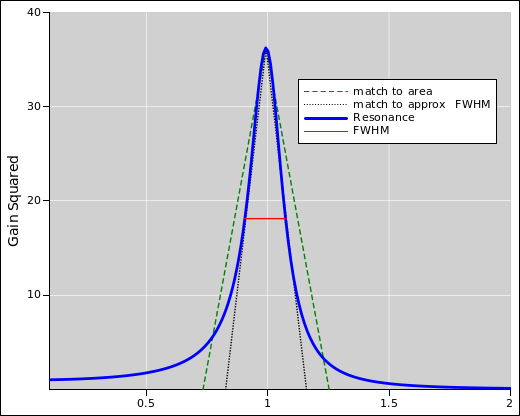Figure 2: Resonance, Q=6, Linear Axes Figure 3: Resonance, Q=6, Linear Axes, Zoomed In

Another possible pitfall is aliasing, as discussed in section 3.2.

### 1.5  Peak Position, Peak Height, and Asymptotes

At the corner frequency the height is:

 1/(ω02 τ2) = 1/(R2C2 / LC) = (L/C) / R2 ω02/δ2 = (1/LC)/(R2/L2) = (L/C) / R2 = Z02/R2 = Q2
(27)

If Q is not very high, the actual peak of the resonance is slightly taller than that, and occurs at a slightly lower frequency; see equation 14 and equation 28.

Also note:

• The gain squared goes to 1 at low frequencies. This is indicated by the dotted line in figure 5.
• It falls off like the fourth power at high frequencies, and is asymptotically equal to (ω0/ω)4. This is indicated by the dotted line in figure 5.
• When the Q is not super-high, the peak of the resonance is slightly below the corner frequency. To a better approximation its at 1 − 1/4/Q2; for details see equation 13c and/or equation 28. The shift is just barely visible in figure 3.

In addition to equation 13, here is another way of locating the peak. Set the derivative of (gain squared) to zero. Then:

0 = (d/dϕ) [(1/Q2) ϕ2 + (ϕ2 − 1)2]
= 2ϕ/Q2 + 2(ϕ2−1) (2ϕ)
0 = ϕ2 + 1/2/Q2 − 1
ϕpeak =
 1 − 1/2/Q2

= 1 − 1/4/Q2 plus terms of order Q−4
(28)

At that frequency, the height of the peak is as follows, as you can verify by direct substitution:

|G|2peak =  4 Q4 4 Q2 − 1
(29)

Both equation 28 and equation 29 agree with the results we obtained in equation 14 by other means.

• The center-of-gravity of the distribution is shifted even more than that, because the wings of the distribution are not symmetrical.
• On the other hand, the corner frequency, i.e. the intersection of the asymptotes, is exactly f0 aka ω0.

The following figures were made using the spreadsheet mentioned in reference 3.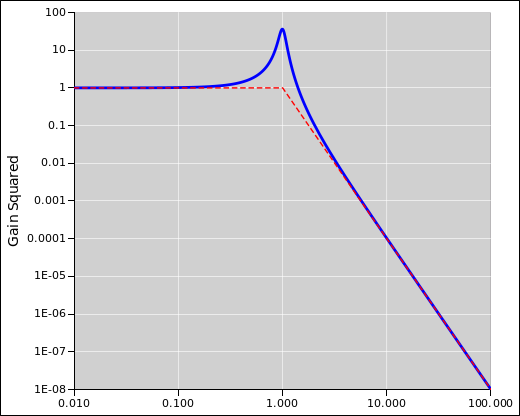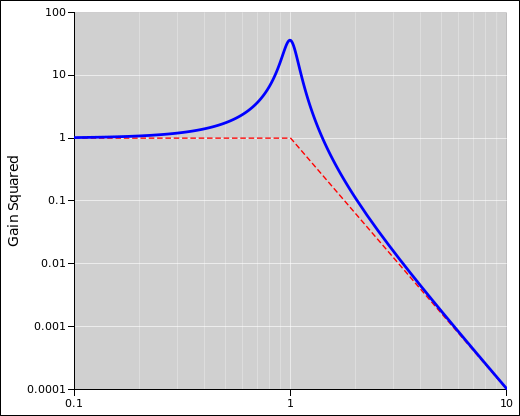Figure 4: Resonance, Q=6 Figure 5: Resonance, Q=6, Zoomed In

### 1.6  Frequency-Like Quantities

• The corner frequency, ω0, is obviously an important quantity. It is equal to the geometric mean of the R/L frequency and the 1/(RC) frequency.

ω0 =
 R L
 1 RC
]½
=
1/ LC
(30)

• The R/L bandwidth is smaller than the corner frequency by a factor of Q, as you can see from equation 7. It gives a good description of the FWHM of the resonant peak, assuming Q is not too small.

 R L
=  ω0 Q
(31)

• In contrast, 1/(RC) is larger than the corner frequency by a factor of Q.

 1 RC
= Q ω0
(32)

It has dimensions of frequency, but it is not what people think of as the bandwidth of the resonance. It could be considered the effective noise bandwidth, if we were rash enough to interpret equation 24 as 4 kT R times some bandwidth, and ignore a factor of 4, and ignore the issue of gain.

A better way to think of 1/(RC) is as a bandwidth times gain squared. The RLC circuit doesn’t just “select” the components of the incoming signal that lie within the passband; it also amplifies those components.

• There is also the frequency corresponding to the actual peak of the resonance. For large Q, this is close to the corner frequency.

### 1.7  Voltages

There are at least five voltages of interest in our circuit:

1. The unobservable ideal noise voltage source VN inside the resistor, inside the black box, as given by the Nyquist formula.
2. The unobservable IR drop across the ideal, noiseless, resistive part of the resistor, inside the black box.
3. The observable voltage VRx at the terminals of the real resistor.
4. The voltage across the inductor.
5. The voltage across the capacitor.

## 2  Derivation – Grounded Inductor

### 2.1  Basic Formulas

This is closely analogous to the analysis in section 1.1.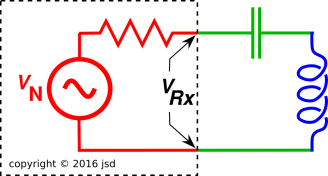Figure 6: Series RLC Circuit, Grounded Inductor

|I0| =
|V0
R2 + (ωL −
 1 ωC
)2 ]½
(33)

The magnitude of voltage across the capacitor is:

|VL| = |I0| |ZL|     (34a)
=
|V0| ω2LC
ωC [ R2 + (ωL −
 1 ωC
)2 ]½
(34b)

so the magnitude of the gain is:

 VL V0

=
ω2LC
[ ω2C2R2 + (ω2LC −
 ωC ωC
)2 ]½
(35a)
=
 ω2/ω02 [ (1/Q2)ω2/ω02 + (ω2/ω02 − 1)2 ]½
(35b)

where as before:

ω0 :=
 1/LC

Q :=
(1/R
 L/C

(36)

Equation 35 says the gain goes to unity at high frequencies. This should be obvious from looking at the circuit diagram. At the resonant frequency, the height of the peak is Q.

## 3  Digital Sampling

We now turn to the “digital signal processing” point of view. That is, we imagine the current, voltage, etc. are represented by time-series data, i.e. a sequence of samples.

This stands in contrast to section 1, which analyzed things from an analog point of view.

### 3.1  Magnitude of the Noise Source

Let the time between samples be Δt.

The thermal noise source, internal to the resistor, should have a mean square voltage:

 ⟨|VN|2⟩ = 4 kT R / Δt / 2 = 4 kT R fN
(37)

where fN is the Nyquist frequency. Compare equation 21.

Here’s one way to understand why fN appears in this expression: Imagine this noise source is driving a filter, and ask how big much bandwidth B is needed in order to capture all of the power coming from this source. That is the meaning of the B used in the derivation of the Nyquist formula.

You can verify that this is correct as follows: Use this voltage to drive a filter. An ultra-simple RC filter suffices. Integrate the equations of motion numerically. Verify that the average thermal energy in the capacitor is ½ kT, independent of all circuit parameters.

### 3.2  Aliasing

The RLC filter responds to waves at negative frequencies just as well as to positive frequencies. That means it looks like the black curve in figure 7. At the Nyquist frequency it is a factor of 2 higher than the blue curve (which is the same as in figure 4). One octave below the Nyquist frequency, it is higher by a few percent.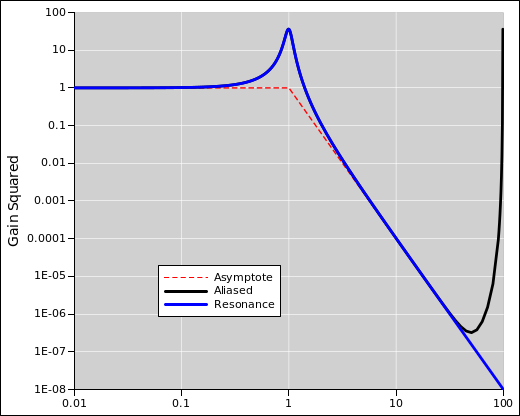Figure 7: Resonance, Q=6, with Aliasing

In this example, the sampling rate is 200 Hz, so the Nyquist frequency is 100 Hz. On linear axes the curve is symmetrical, i.e. an even function of frequency, but the symmetry is lost when we plot it on log axes.

When fitting the data, if you’re in a hurry you might just throw away the top two octaves ... but it’s almost as easy to include the aliasing in the model. Instead of equation 9, use the following:

|G|2 =
 1 (1/Q2) f2/f02 + (f2/f02 − 1)2

+
 1 (1/Q2) g2/f02 + (g2/f02 − 1)2
(38)

where g is the sampling frequency minus f. At the Nyquist frequency, g=f.

Here is some real data, from timestepping the equations of motion for a simple RC (not RLC) circuit. That can be done super-easily.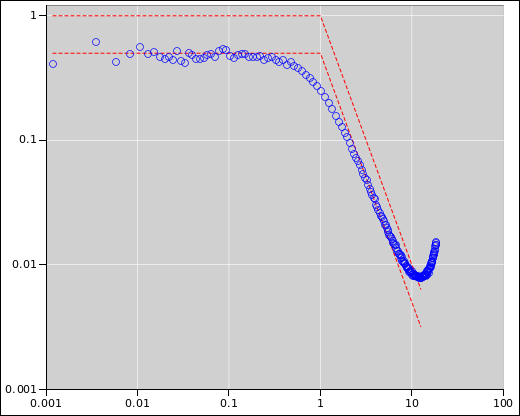Figure 8: FFT View of Noise in an RC Circuit

The corner frequency is 1 Hz, and the Nyquist frequency is 12.5 Hz. The ordinates are normalized to the ideal intrinsic noise in the resistor, so they “should” go to one at low frequency, because the filter has unity gain at low frequencies. The power-spectrum data as reported by the FFT, as shown in the figure, is off by a factor of two, because of aliasing. Half of the energy we are looking for is lurking at negative frequencies. When we look at the FFT we see only positive frequencies, whereas a real hardware RC has no such restriction.

### 3.3  Digital Filter Accuracy

As long as the resonant frequency is small compared to the Nyquist frequency, and you don’t look too closely at the tails of the distribution (as discussed in section 3.2), the results should be independent of the sampling rate.

In practice, digitial filters are always followed by analog filters, to solve the aliasing problem.

In our case, the RLC makes a fine analog filter all by itself, and (if properly used) will filter out 99.99% of the ugliness at negative frequencies and high frequencies.

## 4  References

1.
MIT
http://web.mit.edu/8.02t/www/materials/StudyGuide/guide12.pdf

2.
John Denker,
“Introduction to Probability”
www.av8n.com/physics/probability-intro.htm

3.
John Denker,
“Spreadsheet to Calculate RLC Response Function”
./rlc.xls
./rlc.gnumeric
and the maxima code for checking the calculations:
./rlc.max
[Contents]